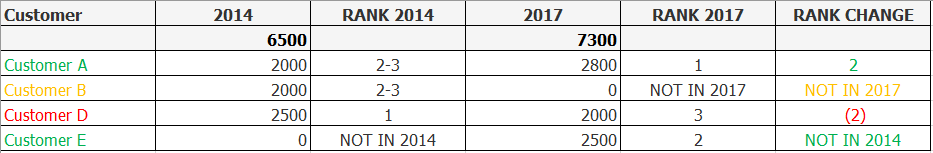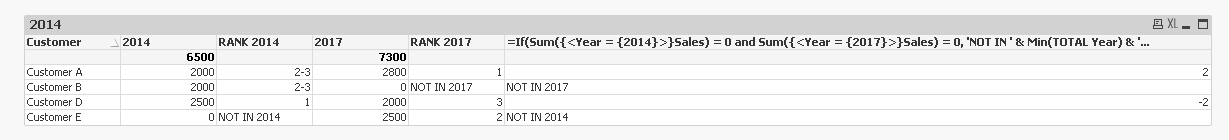# New to QlikView

Discussion board where members can get started with QlikView.

Announcements
Talk to Experts Tuesday, Live Q&A, September 22: Moving from QlikView to Qlik Sense. REGISTER
cancel
Showing results for
Did you mean:
HighlightedContributor II

## Rank Difference Across Years

Hi,

I have sales data of customers across years. I need to get the rank of the customer by the year and then get the difference in the rank between two years. ie. If the customer has moved up or down etc.

I am trying to achieve the output as per the below.Have attached the sample data.

Note: Am using QV 11.20 personal edition thus please post the script and the expressions for the solution as will not be able to access other QVW's.

Thanks.

1 Solution

Accepted Solutions
HighlightedMVP

Here are the new expressions

=Sum({<Year = {\$(vYearFrom)}>}Sales)

=If(Column(1) <> 0 or Column(3) <> 0, If(Sum({<Year = {\$(vYearFrom)}, Customer>}Sales) > 0, Rank(Sum({<Year = {\$(vYearFrom)}, Customer>}Sales), 3), 'NOT IN ' & \$(vYearFrom)))

=Sum({<Year = {\$(vYearTo)}>}Sales)

=If(Column(1) <> 0 or Column(3) <> 0, If(Sum({<Year = {\$(vYearTo)}, Customer>}Sales) > 0, Rank(Sum({<Year = {\$(vYearTo)}, Customer>}Sales), 3), 'NOT IN ' & \$(vYearTo)))

=If(Column(1) <> 0 or Column(3) <> 0, If(SecondaryDimensionality() > 0,

If(Sum({<Customer>}Sales)=0,'NOT IN YEAR',Rank(Sum({<Customer>}Sales))),

If(Sum({<Year = {\$(vYearFrom)}, Customer>} Sales)=0,'NOT IN '&\$(vYearFrom),

If(Sum({<Year = {\$(vYearTo)}, Customer>} Sales)=0,'NOT IN '&\$(vYearTo),

Rank(Sum({<Year={\$(vYearFrom)}, Customer>} Sales),0,1)-Rank(Sum({<Year = {\$(vYearTo)}, Customer>} Sales),0,1)))))

17 Replies
HighlightedMVP

Script

Table:

Year,

Sales

FROM

(ooxml, embedded labels, table is Sheet1);

Straight Table

Dimension

Customer

Expressions

=Sum({<Year = {\$(=Min(Year))}>}Sales)

=If(Sum({<Year = {\$(=Min(Year))}>}Sales) > 0, Rank(Sum({<Year = {\$(=Min(Year))}>}Sales), 3))

=Sum({<Year = {\$(=Max(Year))}>}Sales)

=If(Sum({<Year = {\$(=Max(Year))}>}Sales) > 0, Rank(Sum({<Year = {\$(=Max(Year))}>}Sales), 3))

=Column(2) - Column(4)Note: Attaching the qvw for other visitiors

HighlightedMaster

Hi Cool,

=rank(Sum(Sales),1,1)

Gives this pivot table:

 Customer Year 2014 2015 2016 2017 Customer A 2 1 2 1 Customer B 2 3 2 4 Customer C 4 2 1 4 Customer D 1 3 2 3 Customer E 4 3 2 2

OR

Rank(sum({\$<Year={\$(=-3+max(Year))}>}Sales),1,1)

& '=>' &

Rank(sum({\$<Year={\$(=-2+max(Year))}>}Sales),1,1)

& '=>' &

Rank(sum({\$<Year={\$(=-1+max(Year))}>}Sales),1,1)

& '=>' &

Rank(sum({\$<Year={\$(=max(Year))}>}Sales),1,1)

gives this straight table:

Customer Y to Y
Customer A2=>1=>2=>1
Customer B2=>3=>2=>4
Customer C4=>2=>1=>4
Customer D1=>3=>2=>3
Customer E4=>3=>2=>2

Cheers

Andrew

HighlightedContributor II

Hi Sunny,

Thanks for your reply. I also need to mention 'NOT IN' followed by the YEAR in the chart as per the image attached.

Can you help me get that too.

Thanks.

HighlightedContributor II

Hi Andrew,

Thanks for your reply. I also need to mention 'NOT IN' followed by the YEAR in the chart as per the image attached.

Can you help me get that too.

Also for eg. Customer B has sales only in 2014, thus for what i am trying to do there should be no rank for the customer in the years where there is no sales.

Thanks.

HighlightedMaster III

Hi,

Expressions :

Sum(Sales)

If(SecondaryDimensionality() > 0,
If(Sum(Sales)=0,'NOT IN YEAR',Rank(Sum(Sales))),
If(Sum({<Year={'\$(=Min(Year))'}>} Sales)=0,'NOT IN '&Min(Year),
If(Sum({<Year={'\$(=Max(Year))'}>} Sales)=0,'NOT IN '&Max(Year),
Rank(Sum({<Year={'\$(=Min(Year))'}>} Sales),0,1)-Rank(Sum({<Year={'\$(=Max(Year))'}>} Sales
),0,1))))Regards,

Antonio

HighlightedMaster

Hi Cool,

 Year Customer 2014 2017 Sales Rank Rank change Sales Rank Rank change Customer A 2000 2 2800 1 +1 Customer B 2000 2 0 Not in 2017 -2 Customer D 2500 1 2000 3 -2 Customer E 0 Not in 2014 2500 2 +2

Rank: if(Sum(Sales) >0,rank(Sum(Sales),1,1), 'Not in ' & Year)

Rank change: Before(rank(Sum(Sales),1,1)) - rank(Sum(Sales),1,1)

Format the number return by Rank change to get +/-

good luck

Andrew

HighlightedMVP

Expressions

=Sum({<Year = {\$(=Min(Year))}>}Sales)

=If(Sum({<Year = {\$(=Min(Year))}>}Sales) > 0, Rank(Sum({<Year = {\$(=Min(Year))}>}Sales), 3), 'NOT IN ' & Min(TOTAL Year))

=Sum({<Year = {\$(=Max(Year))}>}Sales)

=If(Sum({<Year = {\$(=Max(Year))}>}Sales) > 0, Rank(Sum({<Year = {\$(=Max(Year))}>}Sales), 3), 'NOT IN ' & Max(TOTAL Year))

=If(Sum({<Year = {\$(=Min(Year))}>}Sales) = 0 and Sum({<Year = {\$(=Max(Year))}>}Sales) = 0, 'NOT IN ' & Min(TOTAL Year) & ' and ' & Max(TOTAL Year),

If(Sum({<Year = {\$(=Min(Year))}>}Sales) = 0, 'NOT IN ' & Min(TOTAL Year),

If(Sum({<Year = {\$(=Max(Year))}>}Sales) = 0, 'NOT IN ' & Max(TOTAL Year), Column(2) - Column(4))))HighlightedContributor II

Hi Antonio,

Thank you for taking the time out and for the solution.

Just one thing. If i pass the two years as variables and not as selections in the list box then the problem i am facing is that Customer B that has no sales in either of the two years that is 2014 & 2017 still shows up in the chart.

There are a lot of customers like this thus i end up having the customer name and 0 as sales for them for both the years.

Also if i select any customer in the list box the rank for the customer changes how can i include AGGR or Total  or another solution in the expression to avoid this.

Thanks again.

HighlightedMaster III

1) Why the behavior sould change ?

2) I think You must achieve this in Script (like TableOfAs)

Regards,

Antonio